# Problem: The figure shows a graph of the output from an AC voltage source. a. What is the maximum voltage  of the source?b. What is the average voltage  of the source?c. What is the root-mean-square voltage of the source?d. What is the period  of the source?e. What is the frequency  of the source?f. What is the angular frequency  of the source?

###### FREE Expert Solution

a.

The maximum voltage is 3 V

b.

Average voltage:

Vavg = 0 V

The average voltage is 0.

80% (168 ratings)###### Problem Details

The figure shows a graph of the output from an AC voltage source.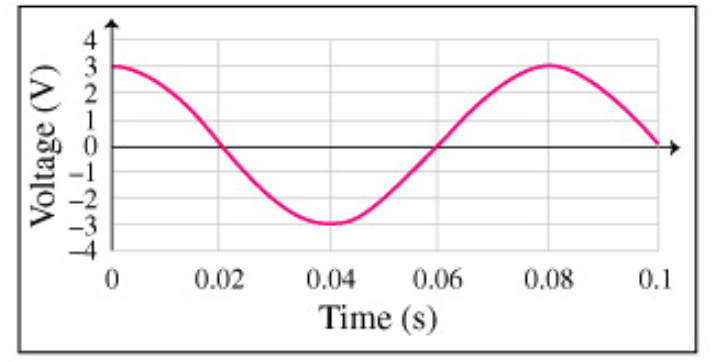a. What is the maximum voltage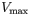of the source?

b. What is the average voltage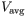of the source?

c. What is the root-mean-square voltage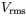of the source?

d. What is the period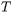of the source?

e. What is the frequency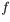of the source?

f. What is the angular frequency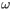of the source?